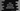# 4 different ways to Sort String characters Alphabetically in Java## Introduction :

Sometimes we need to sort all characters in a string alphabetically. It creates a different string variable since String is immutable in Java. For example, String ‘albert’ will become ‘abelrt’ after sorting. In this Java example, we will learn how to sort the characters of a string alphabetically in different ways.

Let’s take a look :

### Using loop :

The basic way of any sorting is to use loops. We will use two for loops and both will run one inside another. The outer loop will pick one by one element from the left of the string and the inner loop will compare it with all other elements to the left of it. If any element found smaller by the inner loop, we will swap it with the element pointed by the outer loop. Let me show you this algorithm with code :

``````import java.util.Arrays;
import java.util.Scanner;

class Main {
public static void main(String[] args) {
//1
Scanner scanner = new Scanner(System.in);

//2
System.out.println("Enter a string : ");
String userInput = scanner.nextLine();

//3
char[] charArray = userInput.toCharArray();

//4
for (int i = 0; i < charArray.length; i++) {
for (int j = i + 1; j < charArray.length; j++) {
if (Character.toLowerCase(charArray[j]) < Character.toLowerCase(charArray[i])) {
swapChars(i, j, charArray);
}
}
}

//6
System.out.println("Sorted string " + String.valueOf(charArray));
}

//5
private static void swapChars(int i, int j, char[] charArray) {
char temp = charArray[i];
charArray[i] = charArray[j];
charArray[j] = temp;
}

}``````

### Explanation :

1. Create one Scanner object to read the user input values.
2. Ask the user to enter a string. Read it and store it in the userInput variable.
3. We are planning to compare each character of this string and swap and arrange them in ascending order. Since a string is immutable, we need to convert the string value to an array first. For that, we are using toCharArray() that returns an array of characters.
4. Now, sort the contents of the array using two nested for loops. Here, before comparing two characters we are converting them into lowercase since the ASCII values are different for both uppercase and lowercase characters.
5. swapChars function is used to swap two characters in an array. It takes the position of the characters in an array and the array as input.
6. Finally, print out the sorted string to the user.

### Sample Output :

``````Enter a string :
Alphabet
Sorted string Aabehlpt

Enter a string :
elephant
Sorted string aeehlnpt``````

### Sorting without using a loop :

Instead of using two for loops, we can also sort the character array directly like below :

``````import java.util.Arrays;
import java.util.Scanner;

class Main {
public static void main(String[] args) {
Scanner scanner = new Scanner(System.in);

System.out.println("Enter a string : ");
String userInput = scanner.nextLine();

char[] charArray = userInput.toCharArray();

Arrays.sort(charArray);
System.out.println("Sorted string " + String.valueOf(charArray));
}
}``````

The only problem with this method is that it will fail to sort a string with both uppercase and lowercase letters. If the string is only of uppercase or only lowercase, it will work.

### Example :

``````Elephant
Sorted string Eaehlnpt

Enter a string :
elephant
Sorted string aeehlnpt

Enter a string :
ELEPHANT
Sorted string AEEHLNPT``````

### Using a comparator :

We can improve the above program to compare all uppercase and lowercase characters as below :

``````import java.util.Arrays;
import java.util.Comparator;
import java.util.Scanner;

class Main {
public static void main(String[] args) {
Scanner scanner = new Scanner(System.in);

System.out.println("Enter a string : ");
String userInput = scanner.nextLine();

//1
Character[] charArray = new Character[userInput.length()];

for (int i = 0; i < userInput.length(); i++) {
charArray[i] = userInput.charAt(i);
}

//2
Arrays.sort(charArray, Comparator.comparingInt(Character::toLowerCase));

StringBuilder sb = new StringBuilder(charArray.length);
for (Character c : charArray)
sb.append(c.charValue());

System.out.println("Sorted string " + sb.toString());
}
}``````
1. First of all, we are creating one Character array from the string.
2. Then, we have passed one lambda to compare the characters in the second parameter. The lamda is actually like below :
``````Arrays.sort(charArray, new Comparator() {

@Override
public int compare(Character o1, Character o2) {
return Character.compare(Character.toLowerCase(o1),
Character.toLowerCase(o2));
}
});``````

Finally, we are converting the array to string using a StringBuilder. It will arrange the characters as expected.

### Example :

``````Enter a string :
Elephant
Sorted string aEehlnpt``````

### Using Java Stream :

Java 8 stream API provides one beautiful way to solve this problem.

``````import java.util.Comparator;
import java.util.Scanner;
import java.util.stream.Collectors;
import java.util.stream.Stream;

class Main {
public static void main(String[] args) {
Scanner scanner = new Scanner(System.in);

System.out.println("Enter a string : ");
String userInput = scanner.nextLine();

String finalString =
Stream.of(userInput.split(""))
.sorted(Comparator.comparingInt(o -> Character.toLowerCase(o.charAt(0))))
.collect(Collectors.joining());

System.out.println("Sorted string " + finalString);
}
}``````

We are using the same comparator as above inside the sorted() method here. It will give outputs like below :

``````Enter a string :
Elephant
Sorted string aEehlnpt``````

### Conclusion :

We have seen four different ways to sort the characters in a string in Java. Actually, the second and third methods are the same. You can use the third one instead of the second one to support all uppercase and lowercase letters. It is up to your requirement which method you want to use. If you have any doubts or any questions, please drop one comment below.